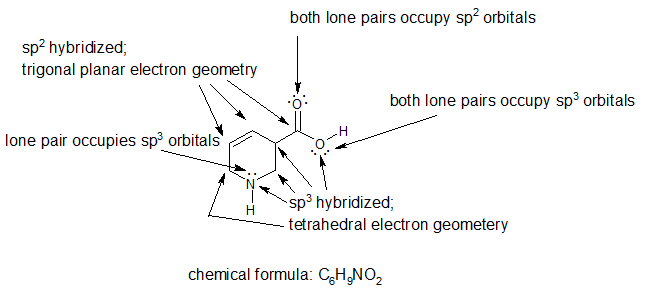# 2.5: Lone Pair Electrons and Bonding Theories

$$\newcommand{\vecs}{\overset { \rightharpoonup} {\mathbf{#1}} }$$ $$\newcommand{\vecd}{\overset{-\!-\!\rightharpoonup}{\vphantom{a}\smash {#1}}}$$$$\newcommand{\id}{\mathrm{id}}$$ $$\newcommand{\Span}{\mathrm{span}}$$ $$\newcommand{\kernel}{\mathrm{null}\,}$$ $$\newcommand{\range}{\mathrm{range}\,}$$ $$\newcommand{\RealPart}{\mathrm{Re}}$$ $$\newcommand{\ImaginaryPart}{\mathrm{Im}}$$ $$\newcommand{\Argument}{\mathrm{Arg}}$$ $$\newcommand{\norm}{\| #1 \|}$$ $$\newcommand{\inner}{\langle #1, #2 \rangle}$$ $$\newcommand{\Span}{\mathrm{span}}$$ $$\newcommand{\id}{\mathrm{id}}$$ $$\newcommand{\Span}{\mathrm{span}}$$ $$\newcommand{\kernel}{\mathrm{null}\,}$$ $$\newcommand{\range}{\mathrm{range}\,}$$ $$\newcommand{\RealPart}{\mathrm{Re}}$$ $$\newcommand{\ImaginaryPart}{\mathrm{Im}}$$ $$\newcommand{\Argument}{\mathrm{Arg}}$$ $$\newcommand{\norm}{\| #1 \|}$$ $$\newcommand{\inner}{\langle #1, #2 \rangle}$$ $$\newcommand{\Span}{\mathrm{span}}$$$$\newcommand{\AA}{\unicode[.8,0]{x212B}}$$

Learning Objective

• identify the orbitals occupied by lone pair electrons

Valence Bond and Molecular Orbital Theories

The table below summarizes the main points of the two complementary bonding theories. Both theories provide different, useful ways of describing molecular structure. We will use both theories and often blend them to analyze and predict chemical structure and reactivity. The bonding theories are reviewed in greater detail in the next two sections.

Comparison of Bonding Theories
Valence Bond Theory Molecular Orbital Theory
considers bonds as localized between one pair of atoms considers electrons delocalized throughout the entire molecule
creates bonds from overlap of atomic orbitals (s, p, d…) and hybrid orbitals (sp, sp2, sp3…) combines atomic orbitals to form molecular orbitals (σ, σ*, π, π*)
forms σ or π bonds creates bonding and antibonding interactions based on which orbitals are filled
predicts molecular shape based on the number of regions of electron density predicts the arrangement of electrons in molecules
needs multiple structures to describe resonance

## Orbitals of Lone Pair Electrons

There are situations in which we will want to integrate molecular orbital and valence bond theories. Identifying the orbitals of lone pair electrons is one situation. Hybridized orbitals create sigma bonds and hold lone pairs. The sigma bonds create the "framework" that holds all the atoms together as a molecule or ion. Un-hybridized p orbitals create pi bonds perpendicular to this sigma framework. In the future, we will learn that some lone pair electrons on heteroatoms of rings can occupy p orbitals to create aromaticity. Stay tuned for upcoming attractions. For the first ten chapters of this text, we will only focus on non-aromatic compounds.

To identify the orbitals of the lone pair electrons in non-aromatic compounds, we can follow a two-step approach.

Step 1: Add any missing lone pair electrons to the heteroatoms (atoms other than carbon and hydrogen).

Step 2: Determine the hybridization of any atoms with lone pairs (heteroatoms). Lone pairs occupy the hybridized orbitals.

Example

To identify the orbitals of the lone pair electrons in the compound below, we will follow the approach above.Step 2: Determine the hybridization of any atom with lone pairs.The lone pairs on each heteroatom occupy the indicated hybridized orbital.

NOTE: These guidelines only apply for non-aromatic compounds. There can be exceptions to these guidelines for some heterocyclic aromatic compounds. These exceptions are fully explained in a later chapter of this text.

Exercise

1. For the compound below:

a) add the lone pair electrons

b) label the hybridization and electron geometry for all non-hydrogen atoms

c) specify the hybridization of the orbital for each lone pair

d) What is the chemical formula of this compound?